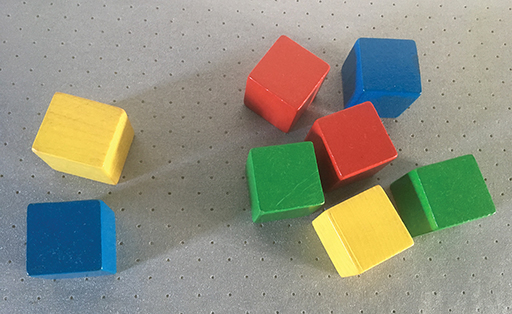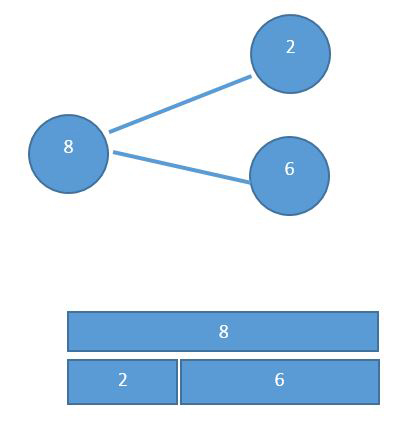Teaching mathematics

Start this free course now. Just create an account and sign in. Enrol and complete the course for a free statement of participation or digital badge if available.

Free course

# 1.3 Part-whole relationships and number bonds

You can make the number 8 in different ways: , , etc. Children can learn these by manipulating physical objects such as bricks and tiles. Starting with 8 objects, a child can split these objects into two or more groups(Figure 3).Figure 3 Using blocks to demonstrate number bonds to 8

This can also be done pictorially using a part-whole diagram or a bar diagram (Figure 4).Figure 4 Diagrams which illustrate

Part-whole relationships are important for learning about number as they develop the understanding of how numbers are made up of other numbers. That is, numbers can be partitioned into smaller constituents in the same way that 8 can be made up of 2 and 6.

The number bonds refer to pairs of numbers which sum to a given number. The important sums are 10, 20, 100, etc. Learning the number bonds to 10 is an important first step in being able to calculate. For example, adding 6 + 7 can be done as 6 + 4 + 3 if it is known that 6 and 4 are numbers which sum to 10. This particular method is known as bridging because you are making the number up to 10 and then adding the remaining part of the number to go past it.

In this way, addition is facilitated by using the number bonds to 10. So, knowing that 4 is added to 6 to make 10, and then knowing the part-whole relationships for 7, i.e. that 7 is also 4 + 3.

TM_1